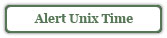# Epoch Time (Unix Time)

Programming often requires representing the world in programming terms. How do we define 'now' in terms of time? We can define it with a date and time, but that becomes very combersome to handle in code. Fortunately there is a way to represent 'now' as an integer. It's called Unix or Epoch time. It is the number of seconds from January 1, 1970. Seem Arbitrary? Maybe, but it gives us a reference point. It has been ?????????? seconds since the epoch or Jan 1, 1970 (UTC).

1/1/1970

## Coordinated Universal Time (UTC)

Unix time is based on the time standard: UTC, which stands for Coordinated Universal Time (which can be confusing because its not abbreviated as you might think) but came about due to a compromise between French (TCU) and English (CUT) spellings of the phrase. Vernacular aside, it is simply a way to describe time without timezones. The reference of January 1, 1970 happened several times across the world, depending on location. Hence the use of of UTC time to define Unix time.

## Accuracy

Okay so it turns out that Unix Time is not exactly the number of seconds from Jan 1, 1970. It's close, but it does not take into account leap seconds. Since the earth's rotation is slowing it takes a little longer to make a full rotation each year. Its less than a second a year but its worth noting.

## Javascript Implementation

To determine the current Unix time in JavaScript we utilize the date class. The getTime method on a date object will return the number of milliseconds since epoch. Let's give it a try:

```var curUnixTime = new Date().getTime();
alert("Number of milliseconds since epoch: " + curUnixTime);```Wait! Technically that is in milliseconds not seconds. To get seconds divide by a thousand and round that bugger so we don't get any decimals points.

```var curUnixTime = Math.round(new Date().getTime() / 1000);
alert("Number of seconds since epoch: " + curUnixTime);```## ActionScript Implementation

ActionScript is almost identical to JavaScript when it comes to Unix time. Again we use the getTime method of the date class. ActionScript's getTime method also returns milliseconds so lets divide those out.

```var curUnixTime:Date = Math.round(new Date().getTime() / 1000);
trace("Number of seconds since epoch: " + curUnixTime);```

## ActionScript Example

Below is an example of converting a date to Unix time. Enter a date, like your birthday, and find out how many seconds it was since the epoch. If the date (or birthday) was before 1970, the number will be negative.

## Possible Problem?

There are a lot of seconds since 1970, and each day 86400 more go by. Eventually we will not be able to store that number in an integer. In most computer systems today a signed 32-bit integer can represent a number up to 2,147,483,647. With a little math we should be able to figure out when this would happen: If a day has 86400 seconds, an average year would have roughly 86,400 * 365 = 31,536,000 seconds. 2,147,483,647 / 31,536,000 = 68.09625 aka 68.1 years. 68 years from 1970 is 2038, hence the term: Year 2038 Problem.